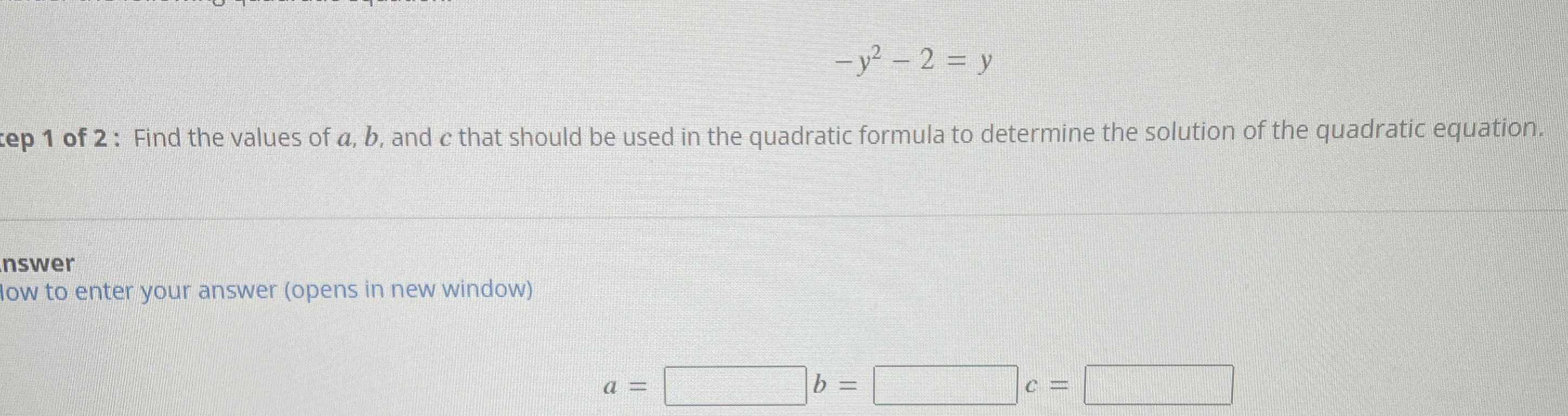### ¿Todavía tienes preguntas de matemáticas?

Pregunte a nuestros tutores expertos
Algebra
Pregunta$$- y ^ { 2 } - 2 = y$$  Find the values of $$a , b$$ , and $$c$$ that should be used in the quadratic formula to determine the solution of the quadratic equation.